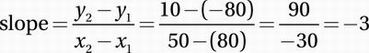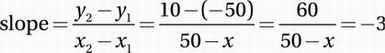# SAT Math Multiple Choice Question 610: Answer and Explanation

### Test Information

Question: 610

10. In the mesosphere, the atmospheric layer between 50 km and 80 km in altitude, the average atmospheric temperature varies linearly with altitude. If the average temperature at 50 km altitude is 10°C and the average temperature at 80 km is -80°C, then at what altitude is the average temperature -50°C?

• A. 60 km
• B. 65 km
• C. 70 km
• D. 75 km

Explanation:

C

Algebra (linear relationships) MEDIUM

We are told that the temperature varies linearly with altitude, so if y represents the temperature (in °C) and x represents altitude (in km), these variables are related by the equation y = mx + b, where m (the slope) and b (the y-intercept) are constants.

We are given two points on this line: (50 km, 10°) and (80 km, -80°). We can use these points to find the slope, m:Recall that the slope of a linear relationship is the "unit rate of change." In other words, the slope of -3 means that the temperature declines by 3° for every 1 km of additional altitude. Since we want the altitude at which the temperature is -50°, we want the value of x such that (x, -50°) is on this line. To find x, we can simply use the slope formula again, using either of the other two points: Slope formula using (50, 10) and (x, -50):Multiply by 50 - x:

60 = -3(50 - x)

Distribute:

60 = -150 + 3x